# 群体固定系数Fst

Fst，用于衡量种群分化程度，取值从0到1，为0则认为两个种群间是随机交配的，基因型完全相似；为1则表示是完全隔离的，完全不相似。它往往从基因的多样性来估计，比如SNP或者microsatellites(串联重复序列一种，长度小于等于10bp)。p1=(125*2+250)/1000=0.5

q1=1-p1=0.5

p2=(50*2+30)/200=0.65

q2=1-p2=0.35

p3=(100*2+500)/2000=0.35

q3=1-p3=0.65

E(Aa)=500*0.5*0.5*2=250 (与实际相等)

E(aa)=500*0.5*0.5=125 (与实际相等)

E(Aa)=100*0.65*0.35*2=45.5 (实际比预期少15.5)

E(aa)=100*0.35*0.35=12.25 (实际比预期多7.75)

E(Aa)=1000*0.35*0.65*2=455 (实际比预期多45个)

E(aa)=1000*0.65*0.65=422.5 (实际比预期少22.5)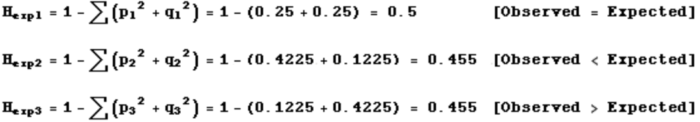F1=(0.5-0.5)/0.5=0 不近交，不远交

F2=(0.455-0.3)/0.455=0.341 存在近交情况，实际杂合子比预期的少

F3=(0.455-0.5)/0.455=-0.099 存在远交情况，实际杂合子比预期的多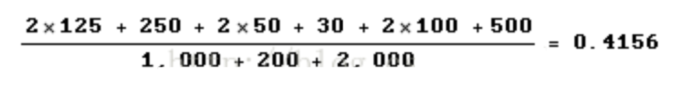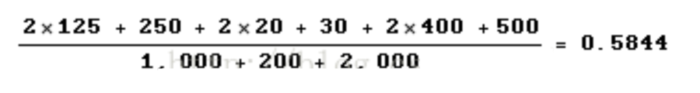HI(实际杂合度)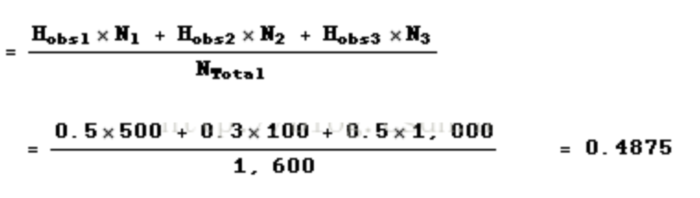HS(亚群预计杂合度--通过每个亚群预计杂合度(亚群等位基因频率)来计算)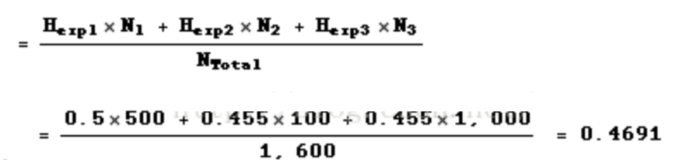HT(种群预计杂合度--通过种群中预计等位基因频率来计算)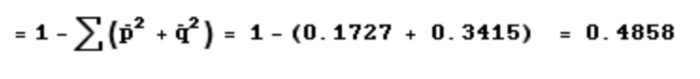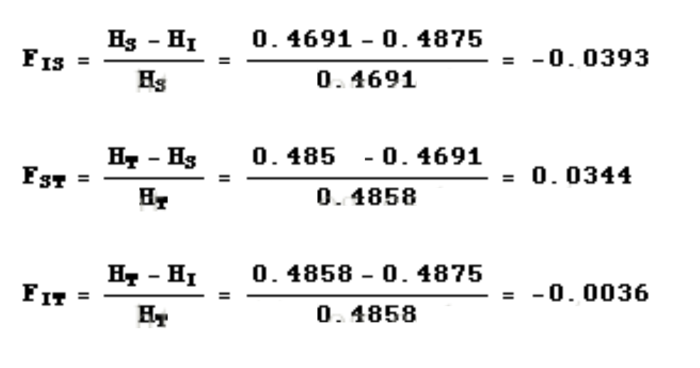• 版权声明 本文源自 -麦_子- 整理 发表
• 转载请务必保留本文链接：https://www.plob.org/article/21653.html